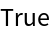#Function Repository Resource:

# CayleyMengerMatrix

Evaluate the Cayley-Menger matrix of a simplex

Contributed by: Jan Mangaldan
 ResourceFunction["CayleyMengerMatrix"][{p1,…,pk}] gives the Cayley-Menger matrix of the simplex spanned by points pi. ResourceFunction["CayleyMengerMatrix"][reg] gives the Cayley-Menger matrix of the region reg.

## Details

The Cayley-Menger matrix of a simplex is the matrix of squared distances between each pair of vertices of the simplex, bordered by 1s at left and on top, and 0 in the upper left corner.
For ResourceFunction["CayleyMengerMatrix"][reg], reg can be any valid Triangle, Tetrahedron or Simplex.

## Examples

### Basic Examples (2)

The Cayley-Menger matrix of a triangle represented as a list of its vertices:

 In:=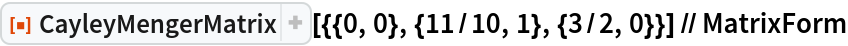Out=The Cayley-Menger matrix of a Triangle:

 In:=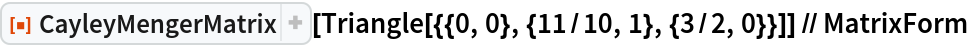Out=### Scope (3)

The Cayley-Menger matrix of a tetrahedron, specified by its vertex list:

 In:=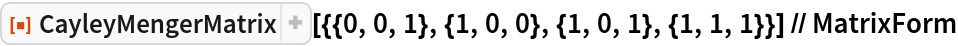Out=The Cayley-Menger matrix of a tetrahedron, specified as a Tetrahedron:

 In:=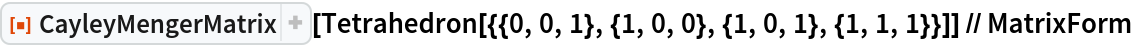Out=The Cayley-Menger matrix of a tetrahedron, specified as a Simplex:

 In:=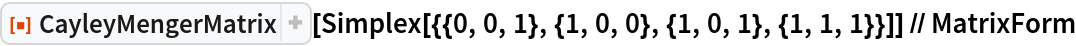Out=### Applications (3)

Use the Cayley-Menger matrix to evaluate the content of a simplex:

 In:=Compute the area of a triangle:

 In:=Out=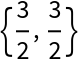Compute the volume of a tetrahedron:

 In:=Out=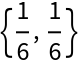Compute the content of a four-dimensional simplex:

 In:=Out=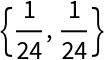Use the Cayley-Menger matrix to obtain the circumsphere of a simplex:

 In:=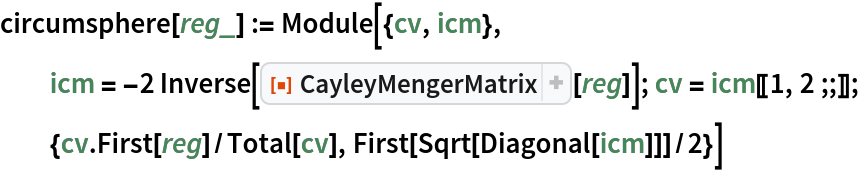Circumcenter and circumradius of a triangle:

 In:=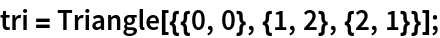In:=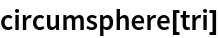Out=Compare with the result of Circumsphere:

 In:=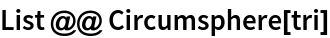Out=Circumcenter and circumradius of a tetrahedron:

 In:=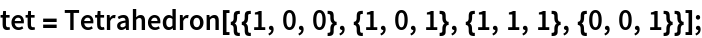In:=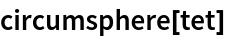Out=Compare with the result of Circumsphere:

 In:=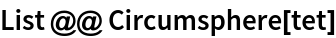Out=Use the Cayley-Menger matrix to obtain the insphere of a simplex:

 In:=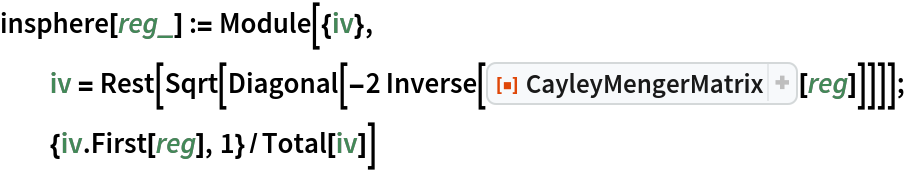Incenter and inradius of a triangle:

 In:=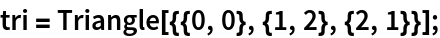In:=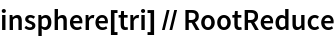Out=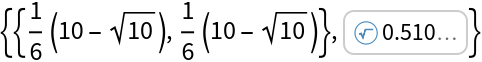Compare with the result of Insphere

 In:=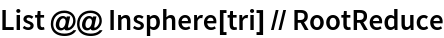Out=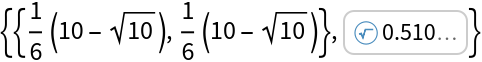Incenter and inradius of a tetrahedron:

 In:=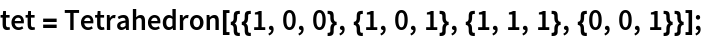In:=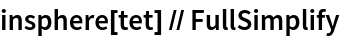Out=Compare with the result of Insphere:

 In:=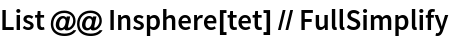Out=### Properties and Relations (1)

The Cayley-Menger matrix is symmetric:

 In:=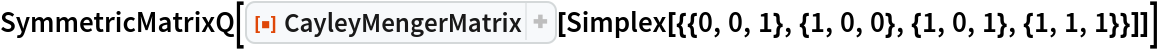Out=